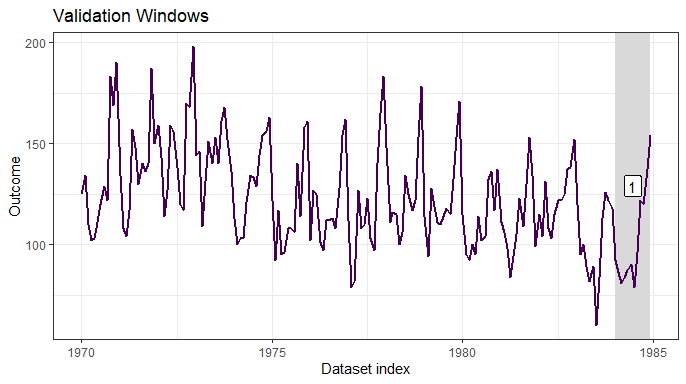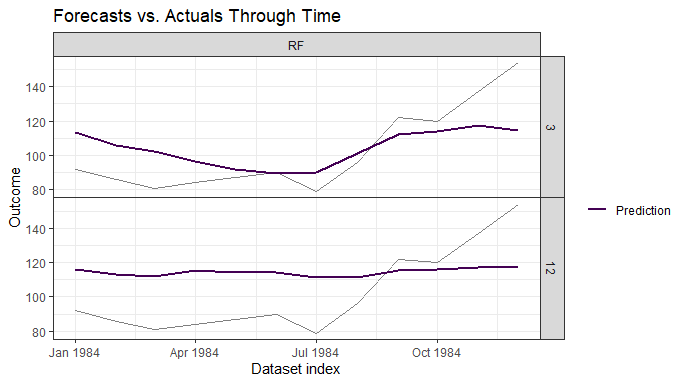# Customizing Wrapper Functions

## Purpose

The purpose of this vignette is to provide a closer look at how the user-supplied model training and predict wrapper functions can be modified to give greater control over the model-building process. The goal is to present examples of how the wrapper functions could be flexibly written to keep a linear workflow in forecastML while modeling across multiple forecast horizons and validation datasets. The alternative would be to train models across a single forecast horizon and/or validation window and customize the wrapper functions for this specific setup.

## Example 1 - Multiple forecast horizons and 1 model training function

• Load packages and data.
library(DT)
library(dplyr)
library(ggplot2)
library(forecastML)
library(randomForest)

data("data_seatbelts", package = "forecastML")
data <- data_seatbelts

data <- data[, c("DriversKilled", "kms", "PetrolPrice", "law")]

dates <- seq(as.Date("1969-01-01"), as.Date("1984-12-01"), by = "1 month")
• Create a lagged data frame for model training. We’ll train 2 models: one across a 1:3-month horizon and the other across a 1:12-month horizon.
data_train <- forecastML::create_lagged_df(data,
type = "train",
outcome_col = 1,
lookback = 1:12,
horizons = c(3, 12),
dates = dates,
frequency = "1 month")

# View the horizon 3 lagged dataset.
DT::datatable(head((data_train$horizon_3)), options = list("scrollX" = TRUE)) • We’ll train our models to predict on 1 validation dataset. Setting window_length = 0 means that a single validation dataset will span from window_start to window_stop. windows <- forecastML::create_windows(data_train, window_length = 0, window_start = as.Date("1984-01-01"), window_stop = as.Date("1984-12-01")) plot(windows, data_train)### User-defined model-training function • The key to customizing training across forecast horizons–here we have 2–is to modify the model training wrapper function based on the horizon-specific dataset in our lagged_df object data_train. • Each dataset’s forecast horizon is stored as an attribute. attributes(data_train$horizon_3)$horizon ##  3 attributes(data_train$horizon_12)$horizon ##  12 • We’ll train a Random Forest model with different settings for the 3-month and 12-month datasets. • The first argument to the user-defined model training function is always the horizon-specific dataset from create_lagged_df(type = "train") and is passed into the wrapper function internally in train_model(). Any number of additional parameters can be defined in this wrapper function by either (a) setting arguments here–like below–or (b) setting the arguments in train_model(...). model_function <- function(data, my_outcome_col = 1, n_tree = c(200, 100)) { outcome_names <- names(data)[my_outcome_col] model_formula <- formula(paste0(outcome_names, "~ .")) if (attributes(data)$horizon == 3) {  # Model 1

model <- randomForest::randomForest(formula = model_formula,
data = data,
ntree = n_tree)

return(list("my_trained_model" = model, "n_tree" = n_tree,
"meta_data" = attributes(data)$horizon)) } else if (attributes(data)$horizon == 12) {  # Model 2

model <- randomForest::randomForest(formula = model_formula,
data = data,
ntree = n_tree)

return(list("my_trained_model" = model, "n_tree" = n_tree,
"meta_data" = attributes(data)$horizon)) } } • Train the models. model_results <- forecastML::train_model(data_train, windows, model_name = "RF", model_function) • View the return() values from the user-defined model_function(). The returned values are stored in my_training_results$horizon_h$window_w$model.
model_results$horizon_3$window_1$model ##$my_trained_model
##
## Call:
##  randomForest(formula = model_formula, data = data, ntree = n_tree)
##                Type of random forest: regression
##                      Number of trees: 200
## No. of variables tried at each split: 13
##
##           Mean of squared residuals: 247.162
##                     % Var explained: 59.72
##
## $n_tree ##  200 ## ##$meta_data
##  3
model_results$horizon_12$window_1$model ##$my_trained_model
##
## Call:
##  randomForest(formula = model_formula, data = data, ntree = n_tree)
##                Type of random forest: regression
##                      Number of trees: 100
## No. of variables tried at each split: 1
##
##           Mean of squared residuals: 420.6497
##                     % Var explained: 31.45
##
## $n_tree ##  100 ## ##$meta_data
##  12

### User-defined prediction function

• The user-defined prediction function only takes two positional parameters. The first parameter is the returned value of the user-defined modeling function; here, a list with 3 elements. The second parameter is the horizon-specific dataset with model features from create_lagged_df() (type = "train" or type = "forecast").
prediction_function <- function(model, data_features) {

if (model$meta_data == 3) { # Perform a transformation specific to model 1. data_pred <- data.frame("y_pred" = predict(model$my_trained_model, data_features))
}

if (model$meta_data == 12) { # Perform a transformation specific to model 2. data_pred <- data.frame("y_pred" = predict(model$my_trained_model, data_features))
}

return(data_pred)
}
• Predict
data_results <- predict(model_results,
prediction_function = list(prediction_function),
data = data_train)
• Plot the performance on the validation dataset.
plot(data_results)